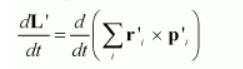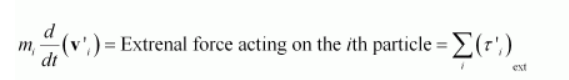# Separation of Motion of a system of particles into motion of the centre of mass and motion about the centre of mass:Question:

Separation of Motion of a system of particles into motion of the centre of mass and motion about the centre of mass:

(a) Show pi = pmiV

Where $\mathbf{p}_{i}$ is the momentum of the $i^{\text {th }}$ particle (of mass $m_{i}$ ) and $\mathbf{p}_{i}{ }^{\prime}=m_{i} \mathbf{v}^{\prime}{ }_{i}$. Note $\mathbf{v}^{\prime}{ }_{i}$ is the velocity of the $i^{\text {th }}$ particle relative to the centre of mass.

Also, prove using the definition of the centre of mass $\sum \mathbf{p}^{\prime}=0$

(b) Show K′ + ½MV2

Where K is the total kinetic energy of the system of particles, K′ is the total kinetic energy of the system when the particle velocities are taken with respect to the centre of mass and MV2/2 is the kinetic energy of the translation of the system as a whole (i.e. of the centre of mass motion of the system). The result has been used in Sec. 7.14.

(c) Show $L=L^{\prime}+R \times M N$

Where $\mathbf{L}^{\prime}=\sum_{i} \mathbf{r}^{\prime}, \times \mathbf{p}^{\prime}$, is the angular momentum of the system about the centre of mass with velocities taken relative to the centre of mass. Remember $\mathbf{r}_{i}=\mathbf{r}_{i}$ - $\mathbf{R}$; rest of the notation is the standard notation used in the chapter. Note $\mathbf{L}^{\prime}$ and $\mathbf{M} \mathbf{R} \times \mathbf{V}$ can be said to be angular momenta, respectively, about and of the centre of mass of the system of particles.

(d) Show $\frac{d \mathbf{L}^{\prime}}{d t}=\sum_{i} \mathbf{r}^{\prime}, \times \frac{d}{d t}\left(\mathbf{p}^{\prime}\right)$

Further, show that

$\frac{d \mathbf{L}^{\prime}}{d t}=\tau_{\mathrm{ext}}^{\prime}$

where $I^{\prime}$ ext is the sum of all external torques acting on the system about the centre of mass.

(Hint: Use the definition of centre of mass and Newton's Third Law. Assume the internal forces between any two particles act along the line joining the particles.)

Solution:

(a)Take a system of moving particles.

Mass of the $i^{\text {th }}$ particle $=m_{i}$

Velocity of the $i^{\text {th }}$ particle $=\mathbf{v}_{i}$

Hence, momentum of the $i^{\text {th }}$ particle, $\mathbf{p}_{i}=m_{i} \mathbf{v}_{i}$

Velocity of the centre of mass $=\mathrm{V}$

The velocity of the $t^{\text {th }}$ particle with respect to the centre of mass of the system is given as:

$\mathbf{v}_{i}^{\prime}=\mathbf{v}_{i}-\mathbf{V}$

$\mathbf{v}_{i}^{\prime}=\mathbf{v}_{i}-\mathbf{v} \ldots(1)$

Multiplying $m_{i}$ throughout equation $(1)$, we get:

$m_{i} \mathbf{v}_{i}^{\prime}=m_{i} \mathbf{v}_{i}-m_{i} \mathbf{v}$

$\mathbf{p}_{i}^{\prime}=\mathbf{p}_{i}-m_{i} \mathbf{V}$

Where,

$\mathbf{p}_{i}{ }^{\prime}=m_{i} \mathbf{v}_{i}{ }^{\prime}=$ Momentum of the $i^{\text {th }}$ particle with respect to the centre of mass of the system

$\therefore \mathbf{p}_{i}=\mathbf{p}_{i}^{\prime}+m_{i} \mathbf{V}$

We have the relation: $\mathbf{p}_{i}{ }^{\prime}=m_{i} \mathbf{v}_{i}{ }^{\prime}$

Taking the summation of momentum of all the particles with respect to the centre of mass of the system, we get:

$\sum_{i} \mathbf{p}_{i}^{\prime}=\sum_{i} m_{i} \mathbf{v}_{i}^{\prime}=\sum_{i} m_{i} \frac{d \mathbf{r}_{i}^{\prime}}{d t}$

Where,

$\mathbf{r}^{\prime},=$ Position vector of $i$ th particle with respect to the centre of mass

$\mathbf{v}_{t}^{\prime}=\frac{d \mathbf{r}^{\prime}}{d t}$

As per the definition of the centre of mass, we have:

$\sum m_{i} \mathbf{r}_{i}^{\prime}=0$

$\therefore \sum_{j} m_{j} \frac{d \mathbf{r}^{\prime},}{d t}=0$

$\sum_{i} p_{1}^{\prime}=0$

(b) We have the relation for velocity of the $i^{\text {th }}$ particle as:

$\mathbf{v}_{i}=\mathbf{v}_{i}^{\prime}+\mathbf{V}$

$\sum_{i} m_{i} \mathbf{v}_{i}=\sum_{i} m_{i} \mathbf{v}_{i}^{\prime}+\sum_{i} m_{i} \mathrm{~V}$

Taking the dot product of equation

$\sum m_{i} \mathbf{v}_{i} \sum m_{i} \mathbf{v}_{i}=\sum m_{i}\left(\mathbf{v}_{;}^{\prime}+\mathbf{v}\right) \cdot \sum m_{i}\left(\mathbf{v}_{i}^{\prime}+\mathbf{v}\right)$

$M^{2} \sum_{i} v_{i}^{2}=M^{2} \sum_{i} v_{i}^{2}+M^{2} \sum_{i} \mathbf{v}_{i} \cdot \mathbf{v}_{i}^{\prime}+M^{2} \sum \mathbf{v}_{i}^{\prime} \cdot \mathbf{v}_{i}+M^{2} V^{2}$

Here, for the centre of mass of the system of particles, $\sum_{i} \mathbf{v}_{i} \mathbf{v}_{,}^{\prime}=-\sum_{i} \mathbf{v}_{i}^{\prime} \mathbf{v}_{i}$

$M^{2} \sum_{i} v_{i}^{2}=M^{2} \sum_{i} v_{i}^{2}+M^{2} V^{2}$

$\frac{1}{2} M \sum v_{i}^{2}=\frac{1}{2} M \sum v_{i}^{\prime 2}+\frac{1}{2} M V^{2}$

$K=K^{\prime}+\frac{1}{2} M V^{2}$

Where,

$K=\frac{1}{2} M \sum v_{i} v_{i}^{2}=$ Total kinetic energy of the system of particles

$K=\frac{1}{2} M \sum_{i} v_{i}^{\prime 2}=$ Total kinetic energy of the system of particles with respect to the centre of mass

$\frac{1}{2} M V^{2}=$ Kinetic energy of the translation of the system as a whole

(c) Position vector of the $t^{\text {th }}$ particle with respect to origin $=\mathbf{r}_{i}$

Position vector of the $i^{\text {th }}$ particle with respect to the centre of mass $=\mathbf{r}_{i}$

Position vector of the centre of mass with respect to the origin $=\mathbf{R}$

It is given that:

$\mathbf{r}_{i}^{\prime}=\mathbf{r}_{j}-\mathbf{R}$

$\mathbf{r}_{i}=\mathbf{r}_{i}{ }_{i}+\mathbf{R}$

We have from part (a),

$\mathbf{p}_{i}=\mathbf{p}_{i}^{\prime}+m_{i} \mathbf{V}$

Taking the cross product of this relation by $\mathbf{r}_{i}$, we get:

$\sum \mathbf{r}_{i} \times \mathbf{p}_{i}=\sum \mathbf{r}_{i} \times \mathbf{p}^{\prime},+\sum_{i} \mathbf{r}_{i} \times m_{j} \mathbf{V}$

$\mathbf{L}=\sum_{i}\left(\mathbf{r}_{i}^{\prime}+\mathbf{R}\right) \times \mathbf{p}_{i}^{\prime}+\sum_{i}\left(\mathbf{r}_{i}^{\prime}+\mathbf{R}\right) \times m_{i} \mathbf{V}$

$=\sum_{i} \mathbf{r}_{i}^{\prime} \times \mathbf{p}_{i}^{\prime}+\sum_{i} \mathbf{R} \times \mathbf{p}^{\prime},+\sum_{i} \mathbf{r}_{i}^{\prime} \times m_{i} \mathbf{V}+\sum_{i} \mathbf{R} \times m_{i} \mathbf{V}$

$=\mathbf{L}^{\prime}+\sum_{i} \mathbf{R} \times \mathbf{p}^{\prime},+\sum_{i} \mathbf{r}^{\prime}, \times m_{j} \mathbf{V}+\sum_{i} \mathbf{R} \times m_{i} \mathbf{V}$

Where,

$\mathbf{R} \times \sum \mathbf{p}_{i}^{\prime}=0$ and

$\left(\sum_{i} \mathbf{r}_{i}^{\prime}\right) \times M \mathbf{V}=\mathbf{0}$

$\sum m_{i}=M$

$\therefore \mathbf{L}=\mathbf{L}^{\prime}+R \times M \mathbf{V}$

(d) We have the relation:

$\mathbf{L}^{\prime}=\sum \mathbf{r}^{\prime}, \times \mathbf{p}^{\prime}$$=\frac{d}{d t}\left(\sum_{i} \mathbf{r}_{\prime}^{\prime}\right) \times \mathbf{p}^{\prime},+\sum_{i} \mathbf{r}_{\prime}^{\prime}, \times \frac{d}{d t}\left(\mathbf{p}_{\prime}^{\prime}\right)$

$=\frac{d}{d t}\left(\sum_{i} m, \mathbf{r}_{\prime}^{\prime}\right) \times \mathbf{v}_{,}^{\prime}+\sum_{i} \mathbf{r}_{,}, \times \frac{d}{d t}\left(\mathbf{p}_{\prime}^{\prime}\right)$

Where, $\mathbf{r}^{\prime}$, is the position vector with respect to the centre of mass of the system of particles.

$\therefore \sum m_{i} \mathbf{r}_{\prime}^{\prime}=0$

$\therefore \frac{d \mathbf{L}^{\prime}}{d t}=\sum_{1} \mathbf{r}_{1}^{\prime} \times \frac{d}{d t}\left(\mathbf{p}_{1}^{\prime}\right)$

We have the relation:

$\frac{d \mathbf{L}^{\prime}}{d t}=\sum_{i} \mathbf{r}^{\prime}, \times \frac{d}{d t}\left(\mathbf{p}^{\prime}\right)$

$=\sum_{i} \mathbf{r}_{i}^{\prime} \times m_{i} \frac{d}{d t}\left(\mathbf{v}_{i}^{\prime}\right)$

Where, $\frac{d}{d t}\left(\mathbf{v}_{i}^{\prime}\right)$ is the rate of change of velocity of the $i$ th particle

with respect of the centre of mass of the system

Therefore, according to Newton's third law of motion, we can write:i.e., $\sum_{i} \mathbf{r}_{i}^{\prime} \times m_{i} \frac{d}{d t}\left(\mathbf{v}_{i}^{\prime}\right)=\tau_{\text {ext }}^{\prime}=$ External torque acting on the system as a whole

$\therefore \frac{d \mathbf{L}^{\prime}}{d t}=\tau_{\mathrm{ext}}^{\prime}$